Ex 5.2

Chapter 5 Class 10 Arithmetic Progressions
Serial order wise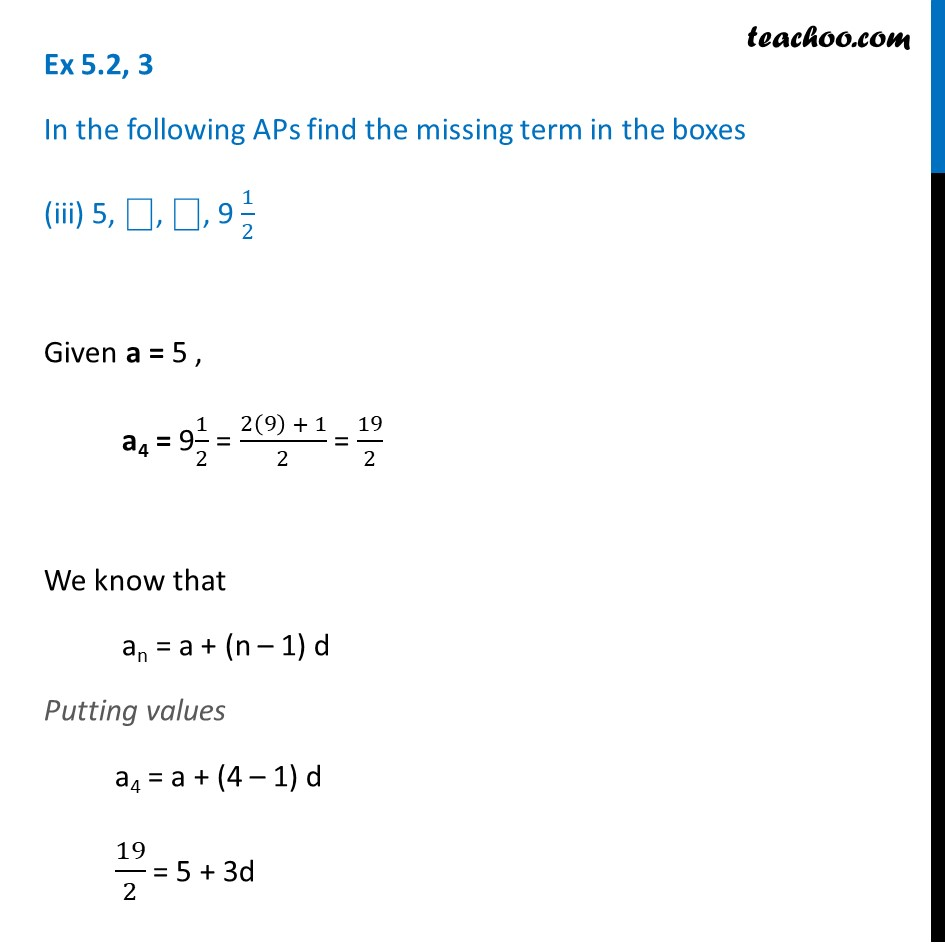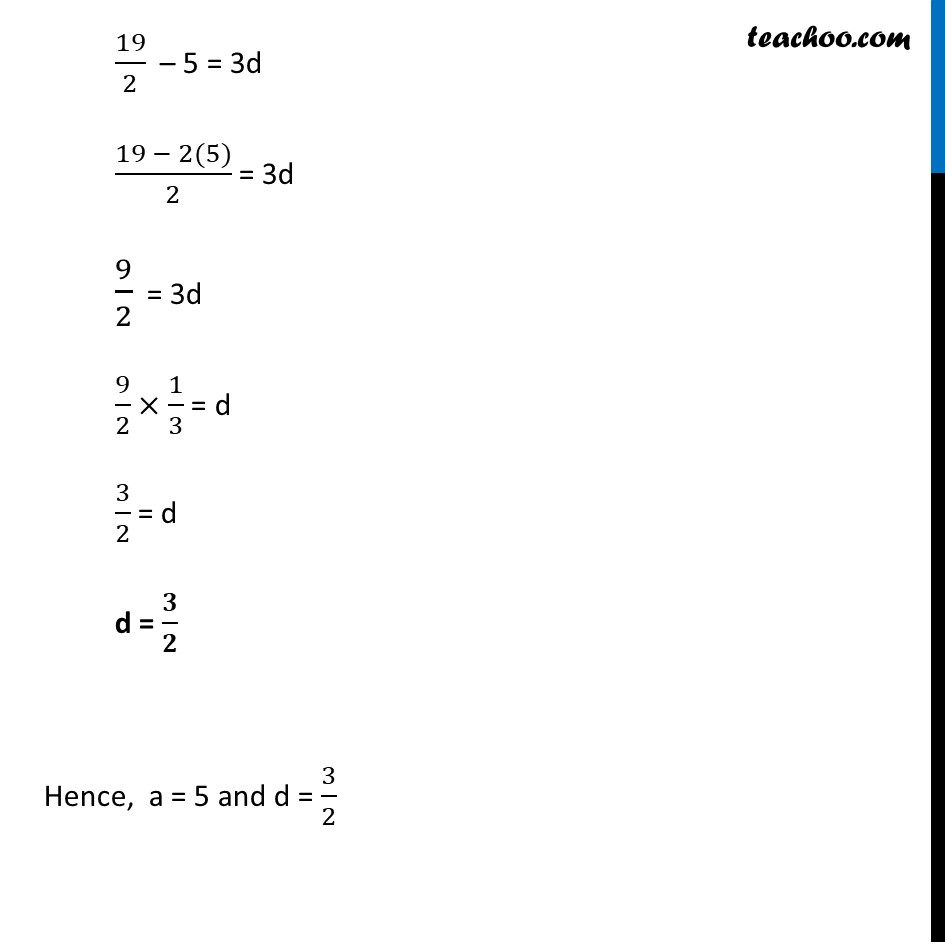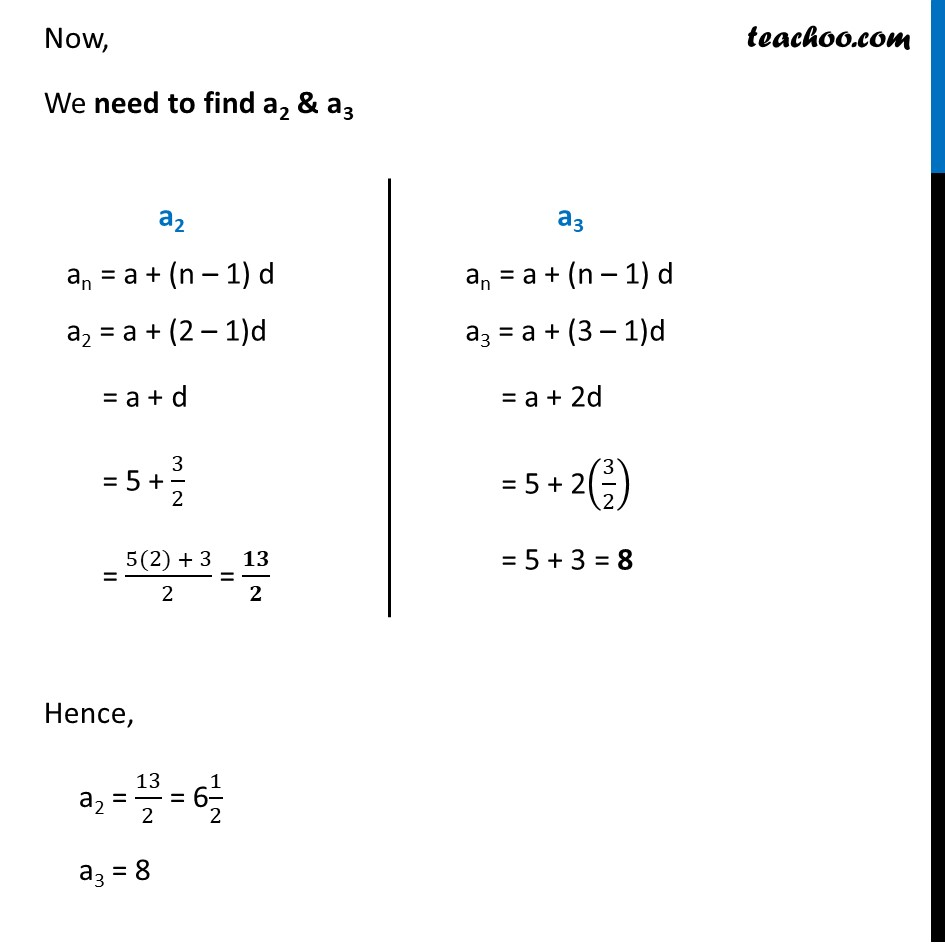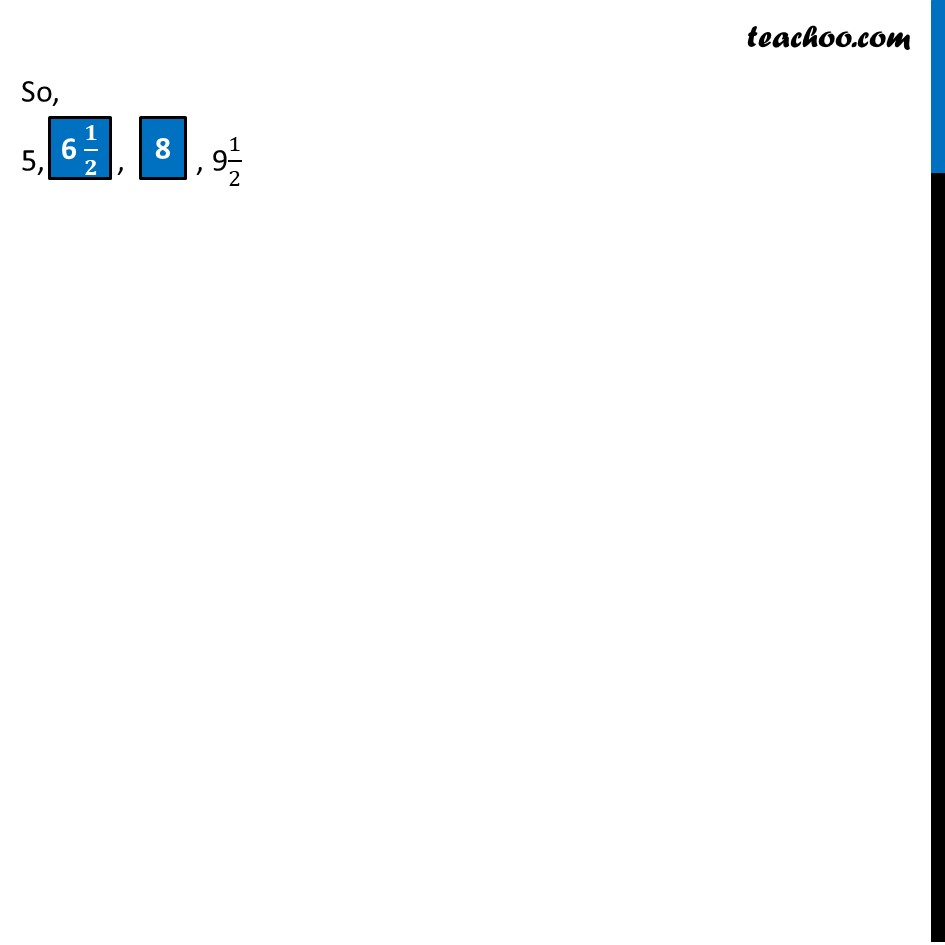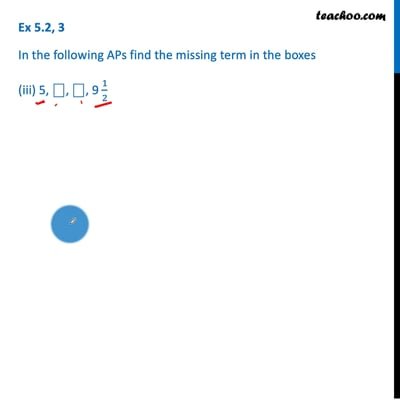This video is only available for Teachoo black users

Introducing your new favourite teacher - Teachoo Black, at only ₹83 per month

### Transcript

Ex 5.2, 3 In the following APs find the missing term in the boxes (iii) 5, ⎕, ⎕, 9 1/2 Given a = 5 , a4 = 91/2 = (2(9) + 1)/2 = 19/2 We know that an = a + (n – 1) d Putting values a4 = a + (4 – 1) d 19/2 = 5 + 3d 19/2 – 5 = 3d (19 − 2(5))/2 = 3d 9/2 = 3d 9/2 × 1/3 = d 3/2 = d d = 𝟑/𝟐 Hence, a = 5 and d = 3/2 Now, We need to find a2 & a3 Hence, a2 = 13/2 = 61/2 a3 = 8 a2 an = a + (n – 1) d a2 = a + (2 – 1)d = a + d = 5 + 3/2 = (5(2) + 3)/2 = 𝟏𝟑/𝟐 a3 an = a + (n – 1) d a3 = a + (3 – 1)d = a + 2d = 5 + 2(3/2) = 5 + 3 = 8 So, 5, , , 91/2